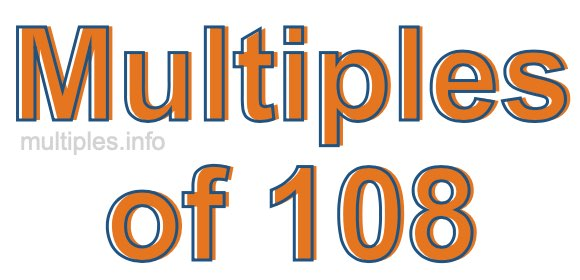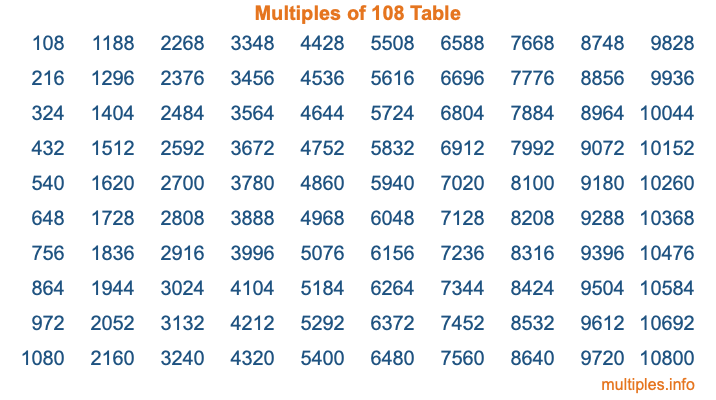Multiples of 108Welcome to the Multiples of 108 page. Here we will first teach you everything you will ever need to know about the multiples of 108, and then give you a study guide summary of everything we taught you to make sure you remember it all. Use this page to look up facts and learn information about the multiples of 108. This page will make you a multiples of one hundred eight expert!

Definition of Multiples of 108
Multiples of 108 are all the numbers that when divided by 108 equal an integer. Each of the multiples of 108 are called a multiple. A multiple of 108 is created by multiplying 108 by an integer.

Therefore, to create a list of multiples of 108, you start with 1 multiplied by 108, then 2 multiplied by 108, then 3 multiplied by 108, and so on for as long as you want. Thus, the list of the first five multiples of 108 is 108, 216, 324, 432, and 540. To see a larger list of multiples of 108, see the printable image of Multiples of 108 further down on this page. We also have a category where you can choose any nth multiple of 108.

Multiples of 108 Checker
The Multiples of 108 Checker below checks to see if any number of your choice is a multiple of 108. In other words, it checks to see if there is any number (integer) that when multiplied by 108 will equal your number. To do that, we divide your number by 108. If the the quotient is an integer, then your number is a multiple of 108.

Is  a multiple of 108?

Least Common Multiple of 108 and ...
A Least Common Multiple (LCM) is the lowest multiple that two or more numbers have in common. This is also called the smallest common multiple or lowest common multiple and is useful to know when you are adding our subtracting fractions. Enter one or more numbers below (108 is already entered) to find the LCM.

Check out our LCM Calculator if you need more details about the Least Common Multiple or if you need the LCM for different numbers for adding and subtraction fractions.

nth Multiple of 108
As we stated above, 108 is the first multiple of 108, 216 is the second multiple of 108, 324 is the third multiple of 108, and so on. Enter a number below to find the nth multiple of 108.

th multiple of 108

Multiples of 108 vs Factors of 108
108 is a multiple of 108 and a factor of 108, but that is where the similarities end. All postive multiples of 108 are 108 or greater than 108. All positive factors of 108 are 108 or less than 108.

Below is the beginning list of multiples of 108 and the factors of 108 so you can compare:

Multiples of 108: 108, 216, 324, 432, 540, etc.

Factors of 108: 1, 2, 3, 4, 6, 9, 12, 18, 27, 36, 54, 108

As you can see, the multiples of 108 are all the numbers that you can divide by 108 to get a whole number. The factors of 108, on the other hand, are all the whole numbers that you can multiply by another whole number to get 108.

It's also interesting to note that if a number (x) is a factor of 108, then 108 will also be a multiple of that number (x).

Multiples of 108 vs Divisors of 108
The divisors of 108 are all the integers that 108 can be divided by evenly. Below is a list of the divisors of 108.

Divisors of 108: 1, 2, 3, 4, 6, 9, 12, 18, 27, 36, 54, 108

The interesting thing to note here is that if you take any multiple of 108 and divide it by a divisor of 108, you will see that the quotient is an integer.

Multiples of 108 Table
Below is an image of the first 100 multiples of 108 in a table. The table is in chronological order, column by column. The first column has the first ten multiples of 108, the second column has the next ten multiples of 108, and so on.The Multiples of 108 Table is also referred to as the 108 Times Table or Times Table of 108. You are welcome to print out our table for your studies.

Negative Multiples of 108
Although not often discussed or needed in math, it is worth mentioning that you can make a list of negative multiples of 108 by multiplying 108 by -1, then by -2, then by -3, and so on, to get the following list of negative multiples of 108:

-108, -216, -324, -432, -540, etc.

Multiples of 108 Summary
Below is a summary of important Multiples of 108 facts that we have discussed on this page. To retain the knowledge on this page, we recommend that you read through the summary and explain to yourself or a study partner why they hold true.

There are an infinite number of multiples of 108.

A multiple of 108 divided by 108 will equal a whole number.

108 divided by a factor of 108 equals a divisor of 108.

The nth multiple of 108 is n times 108.

The largest factor of 108 is equal to the first positive multiple of 108.

108 is a multiple of every factor of 108.

108 is a multiple of 108.

A multiple of 108 divided by a divisor of 108 equals an integer.

108 divided by a divisor of 108 equals a factor of 108.

Any integer times 108 will equal a multiple of 108.

Multiples of a Number
Here you can get the multiples of another number, all with the same attention to detail as we did for multiples of 108 on this page.

Multiples of
Multiples of 109
Did you find our page about multiples of one hundred eight educational? Do you want more knowledge? Check out the multiples of the next number on our list!